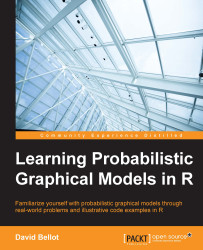•#### Learning Probabilistic Graphical Models in R#### Overview of this book

Probabilistic graphical models (PGM, also known as graphical models) are a marriage between probability theory and graph theory. Generally, PGMs use a graph-based representation. Two branches of graphical representations of distributions are commonly used, namely Bayesian networks and Markov networks. R has many packages to implement graphical models. We’ll start by showing you how to transform a classical statistical model into a modern PGM and then look at how to do exact inference in graphical models. Proceeding, we’ll introduce you to many modern R packages that will help you to perform inference on the models. We will then run a Bayesian linear regression and you’ll see the advantage of going probabilistic when you want to do prediction. Next, you’ll master using R packages and implementing its techniques. Finally, you’ll be presented with machine learning applications that have a direct impact in many fields. Here, we’ll cover clustering and the discovery of hidden information in big data, as well as two important methods, PCA and ICA, to reduce the size of big problems.
Learning Probabilistic Graphical Models in RCreditswww.PacktPub.comPrefaceFree Chapter
Probabilistic ReasoningExact InferenceLearning ParametersBayesian Modeling – Basic ModelsApproximate InferenceBayesian Modeling – Linear ModelsProbabilistic Mixture ModelsAppendixIndex## The Naive Bayes model

The Naive Bayes model is one of the most well-known classification models used in machine learning. Despite its simple appearance, this model is very powerful and gives good results with little effort. Of course, when considering the problem of classification, one should not always stay with one model, such as Naive Bayes, but should try out many examples to see which one is the best with a particular dataset.

Classification is an important problem in machine learning and it could be defined as the task of associating observations to a particular class. Let's say we have a dataset with n variables and we assign a class to each data point. The class could be {0,1} or {a,b,c,d}, {red, blue, green, yellow}, or {warm, cold}, and so on. We will see that it is sometimes easier to consider binary classification problems where one has only two classes. But most classification models can be extended to more than two classes.

For example, given physiological characteristics, we...